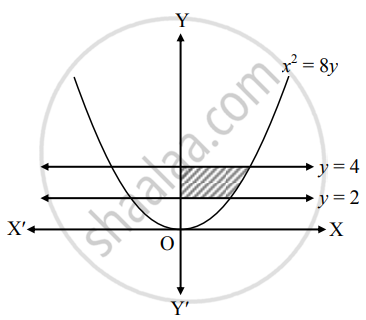# Find the area of the region bounded by the curves x2 = 8y, y = 2, y = 4 and the Y-axis, lying in the first quadrant - Mathematics and Statistics

Sum

Find the area of the region bounded by the curves x2 = 8y, y = 2, y = 4 and the Y-axis, lying in the first quadrant

#### Solution

Given equation of the parabola is x2 = 8y

∴ x = +-  2 sqrt(2y)

∴ x = 2sqrt(2y)     .....[∵ In first quadrant, x > 0]∴ Required area = int_2^4 x  "d"y

= int_2^4 2sqrt(2y)  "d"y

= 2sqrt(2)[(y^(3/2))/(3/2)]_2^4

= (4sqrt(2))/3 [(4)^(3/2) - (2)^(3/2)]

= (4sqrt(2))/3 (8 - 2sqrt(2))

= (8sqrt(2))/3 (4 - sqrt(2)) sq.units

Concept: Area Bounded by the Curve, Axis and Line
Is there an error in this question or solution?